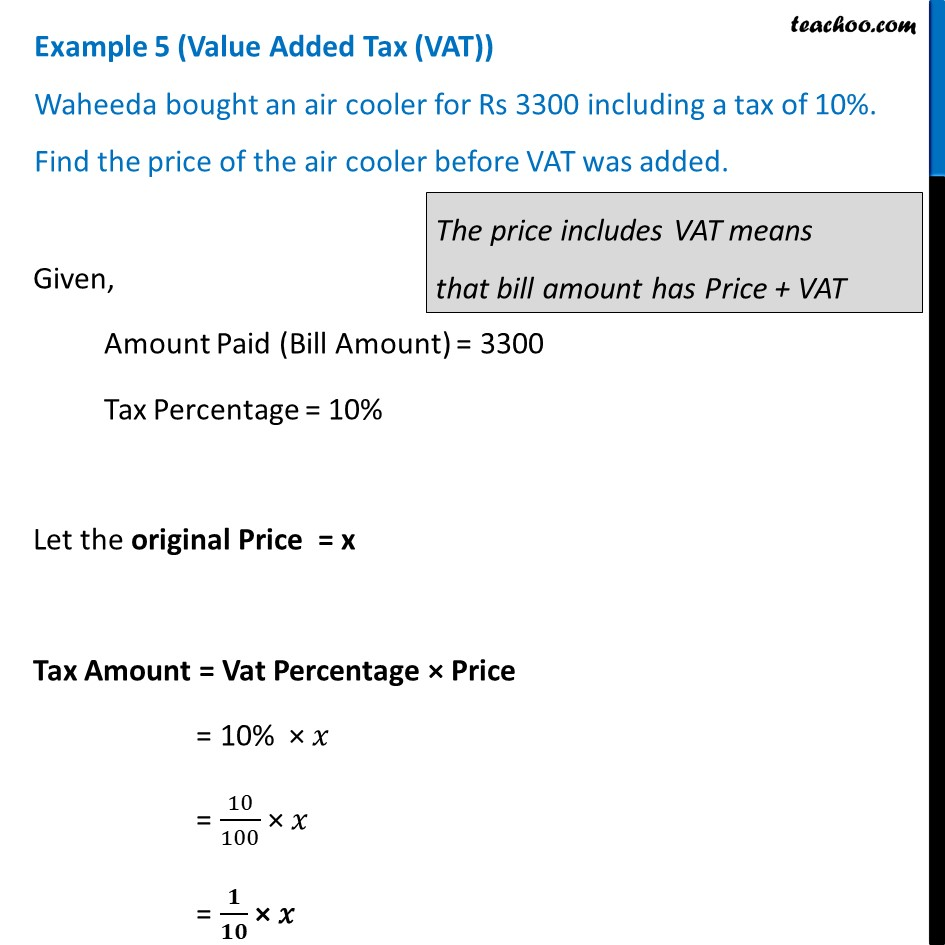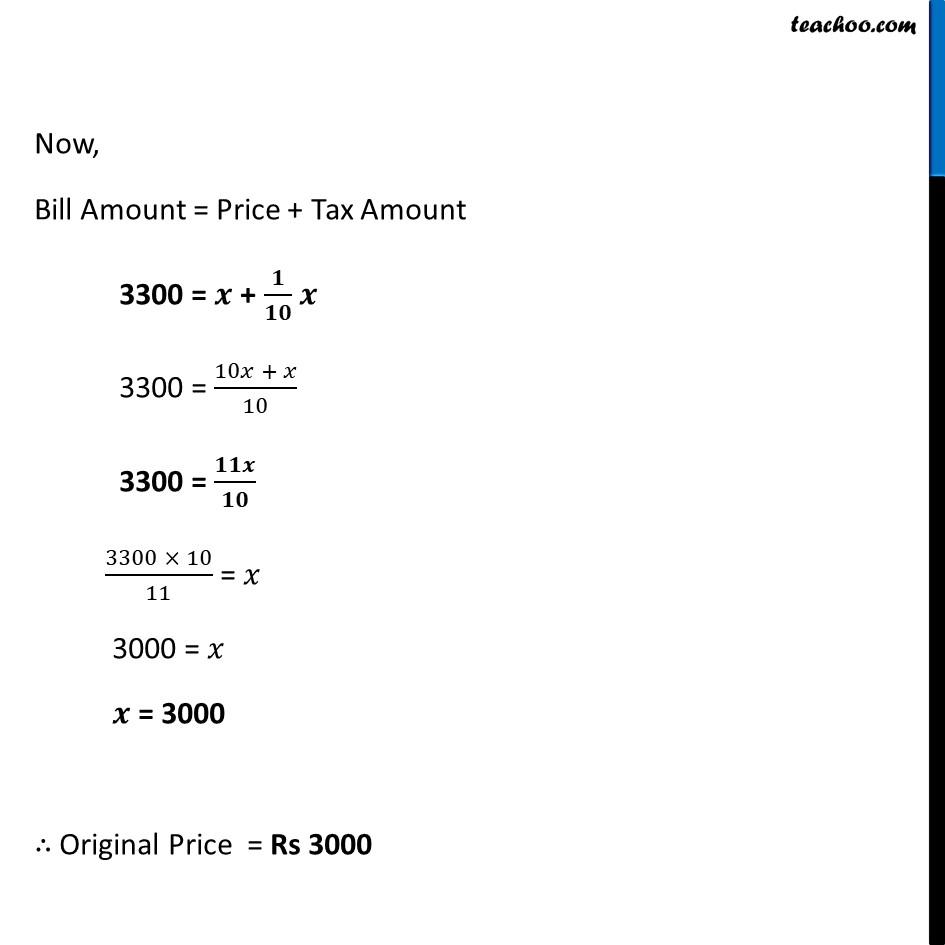Examples

Chapter 7 Class 8 Comparing Quantities
Serial order wiseLearn in your speed, with individual attention - Teachoo Maths 1-on-1 Class

### Transcript

Example 5 (Value Added Tax (VAT)) Waheeda bought an air cooler for Rs 3300 including a tax of 10%. Find the price of the air cooler before VAT was added. Given, Amount Paid (Bill Amount) = 3300 Tax Percentage = 10% Let the original Price = x Tax Amount = Vat Percentage × Price = 10% × 𝑥 = 10/100 × 𝑥 = 𝟏/𝟏𝟎 × 𝑥 Now, Bill Amount = Price + Tax Amount 3300 = 𝒙 + 𝟏/𝟏𝟎 𝒙 3300 = (10𝑥 + 𝑥)/10 3300 = 𝟏𝟏𝒙/𝟏𝟎 (3300 × 10)/11 = 𝑥 3000 = 𝑥 𝒙 = 3000 ∴ Original Price = Rs 3000